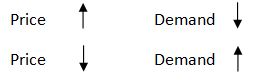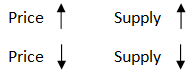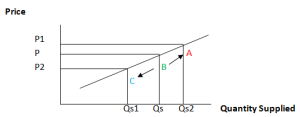Friday , May 24 2019
Home » Igcse Economics Revision Notes » Demand And Supply – How Prices Are Determined

# Demand And Supply – How Prices Are Determined

Prices are determined through the forces of demand and supply of a product or service.

### Demand:

It is the willingness and ability to buy a product / services at a given price over a given period of time.

### Law Of Demand:

The law of demand states that price is inversely proportional to demand.

That means if price increases demand decreases and vice versa if price decreases demand increases.### Demand Curve:An Extension in the Demand Curve: a rise in the quantity demanded caused by a fall in the price of the product itself e.g. (in the above figure from B to C).

A Contraction in the Demand Curve: a fall in the quantity demanded caused by a rise in the price of the product itself e.g. (in the above figure from B to A).

### Supply:

It is the willingness and the ability to sell a good or service at a given price over a given period of time.

### Law of Supply:

The law of supply states that price is directly proportional to the supply of a product .

That means if price increases supply increases and vice versa if price decreases supply decreases.### Supply Curve:An Extension In the Supply Curve: a rise in the quantity supplied caused by a rise in the price of the product itself (B to A).

A Contraction In the Supply Curve: a fall in the quantity supplied caused by a fall in the price of the product itself (B to C).

### Equilibrium Price:

Price where Demand and Supply of a product / service are equal. Thus, there are no shortages or surpluses of the product. It occurs where the demand and supply curves intersect.### Disequilibrium:

Situation where demand and supply are not equal. If the price is above the equilibrium price (P1), that would cause a situation of surplus and if the price of the product is below the equilibrium price (P2), that would cause shortages.

If prices are not at the equilibrium level initially, market forces will move it towards the equilibrium.

### About Admin## Characteristics And Functions Of Money

Money: Money is an item which is generally acceptable as a means of payment. Characteristics …

### One comment

1.Thank you soooo much.

These notes are really helpful